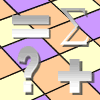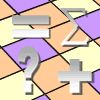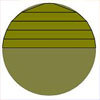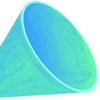Search by Topic

Resources tagged with Spreadsheets similar to Triangles in Circles:

Filter by: Content type:
Age range:
Challenge level:

There are 61 results

Broad Topics > Information and Communications Technology > SpreadsheetsExcel Interactive Resource: Multiples Chain

Age 11 to 16 Challenge Level:

Use an interactive Excel spreadsheet to investigate factors and multiples.Excel Interactive Resource: Interactive Division

Age 11 to 16 Challenge Level:

Use an Excel to investigate division. Explore the relationships between the process elements using an interactive spreadsheet.Excel Interactive Resource: Long Multiplication

Age 11 to 16 Challenge Level:

Use an Excel spreadsheet to explore long multiplication.Excel Interactive Resource: Fraction Multiplication

Age 11 to 16 Challenge Level:

Use Excel to explore multiplication of fractions.Excel Interactive Resource: Equivalent Fraction Bars

Age 11 to 16 Challenge Level:

A simple file for the Interactive whiteboard or PC screen, demonstrating equivalent fractions.Sending a Parcel

Age 11 to 14 Challenge Level:

What is the greatest volume you can get for a rectangular (cuboid) parcel if the maximum combined length and girth are 2 metres?Excel Interactive Resource: the up and Down Game

Age 11 to 16 Challenge Level:

Use an interactive Excel spreadsheet to explore number in this exciting game!Excel Interactive Resource: Number Grid Functions

Age 11 to 16 Challenge Level:

Use Excel to investigate the effect of translations around a number grid.Excel Interactive Resource: Haitch

Age 11 to 16 Challenge Level:

An Excel spreadsheet with an investigation.Percentages for Key Stage 4

Age 14 to 16 Challenge Level:

A group of interactive resources to support work on percentages Key Stage 4.Excel Interactive Resource: Fraction Addition & Fraction Subtraction

Age 11 to 16 Challenge Level:

Use Excel to practise adding and subtracting fractions.Under the Ribbon

Age 14 to 16 Challenge Level:

A ribbon is nailed down with a small amount of slack. What is the largest cube that can pass under the ribbon ?Litov's Mean Value Theorem

Age 11 to 14 Challenge Level:

Start with two numbers and generate a sequence where the next number is the mean of the last two numbers...Substitution Cipher

Age 11 to 14 Challenge Level:

Find the frequency distribution for ordinary English, and use it to help you crack the code.The Fire-fighter's Car Keys

Age 14 to 16 Challenge Level:

A fire-fighter needs to fill a bucket of water from the river and take it to a fire. What is the best point on the river bank for the fire-fighter to fill the bucket ?.Cola Can

Age 11 to 14 Challenge Level:

An aluminium can contains 330 ml of cola. If the can's diameter is 6 cm what is the can's height?Odd Differences

Age 14 to 16 Challenge Level:

The diagram illustrates the formula: 1 + 3 + 5 + ... + (2n - 1) = n² Use the diagram to show that any odd number is the difference of two squares.Age 14 to 16 Challenge Level:

If a sum invested gains 10% each year how long before it has doubled its value?Training Schedule

Age 14 to 16 Challenge Level:

The heptathlon is an athletics competition consisting of 7 events. Can you make sense of the scoring system in order to advise a heptathlete on the best way to reach her target?Euler's Squares

Age 14 to 16 Challenge Level:

Euler found four whole numbers such that the sum of any two of the numbers is a perfect square...What's the Weather Like?

Age 11 to 14 Challenge Level:

With access to weather station data, what interesting questions can you investigate?Pythagoras’ Comma

Age 14 to 16 Challenge Level:

Using an understanding that 1:2 and 2:3 were good ratios, start with a length and keep reducing it to 2/3 of itself. Each time that took the length under 1/2 they doubled it to get back within range.Time of Birth

Age 11 to 14 Challenge Level:

A woman was born in a year that was a square number, lived a square number of years and died in a year that was also a square number. When was she born?Excel Investigation: Pythagorean Triples

Age 11 to 16 Challenge Level:

Use Excel to find sets of three numbers so that the sum of the squares of the first two is equal to the square of the third.Take Ten Sticks

Age 11 to 16 Challenge Level:

Take ten sticks in heaps any way you like. Make a new heap using one from each of the heaps. By repeating that process could the arrangement 7 - 1 - 1 - 1 ever turn up, except by starting with it?Small Change

Age 11 to 14 Challenge Level:

In how many ways can a pound (value 100 pence) be changed into some combination of 1, 2, 5, 10, 20 and 50 pence coins?Excel Technique: Composite Bar Charts

Age 11 to 16 Challenge Level:

Learn how to use composite bar charts in Excel.Excel Investigation: Ring on a String

Age 11 to 16 Challenge Level:

This investigation uses Excel to optimise a characteristic of interest.Minus One Two Three

Age 14 to 16 Challenge Level:

Substitute -1, -2 or -3, into an algebraic expression and you'll get three results. Is it possible to tell in advance which of those three will be the largest ?Tree Tops

Age 14 to 16 Challenge Level:

Can you make sense of information about trees in order to maximise the profits of a forestry company?Excel Interactive Resource: Make a Copy

Age 11 to 16 Challenge Level:

Investigate factors and multiples using this interactive Excel spreadsheet. Use the increment buttons for experimentation and feedback.Peeling the Apple or the Cone That Lost Its Head

Age 14 to 16 Challenge Level:

How much peel does an apple have?Excel Technique: Making a Table for a Function of Two Independent

Age 11 to 16 Challenge Level:

Learn how to make a simple table using Excel.Zeller's Birthday

Age 14 to 16 Challenge Level:

What day of the week were you born on? Do you know? Here's a way to find out.Peaches in General

Age 14 to 16 Challenge Level:

It's like 'Peaches Today, Peaches Tomorrow' but interestingly generalized.Unusual Long Division - Square Roots Before Calculators

Age 14 to 16 Challenge Level:

However did we manage before calculators? Is there an efficient way to do a square root if you have to do the work yourself?Funnel

Age 14 to 16 Challenge Level:

A plastic funnel is used to pour liquids through narrow apertures. What shape funnel would use the least amount of plastic to manufacture for any specific volume ?Excel Investigation: More Beads

Age 11 to 16 Challenge Level:

A heap of beads was shared out by a professional bead sharer. Use the information given to find out how many beads there were at the start.Excel Technique: Inserting an Increment Button

Age 11 to 16 Challenge Level:

Learn how to use increment buttons and scroll bars to create interactive Excel resources.Negative Power

Age 14 to 16 Challenge Level:

What does this number mean ? Which order of 1, 2, 3 and 4 makes the highest value ? Which makes the lowest ?Excel Investigation: Difference Tuples

Age 11 to 16 Challenge Level:

Use an Excel spreadsheet to investigate differences between four numbers. Which set of start numbers give the longest run before becoming 0 0 0 0?Excel Investigation: Multiples of Three

Age 11 to 16 Challenge Level:

Use Excel to investigate digit sums of multiples of three. Can you explain your findings?The Legacy

Age 14 to 16 Challenge Level:

Your school has been left a million pounds in the will of an ex- pupil. What model of investment and spending would you use in order to ensure the best return on the money?Age 11 to 16 Challenge Level:

Use Excel to investigate remainders and divisors. Was your result predictable?Excel Technique: LCM & HCF (or GCD)

Age 11 to 16 Challenge Level:

Learn how to use the Excel functions LCM and GCD.Excel Technique: Using Paste Special to Lift a Copy of Values

Age 11 to 16 Challenge Level:

Learn how to use advanced pasting techniques to create interactive spreadsheets.Excel Investigation: Sums of Consecutive Numbers

Age 11 to 16 Challenge Level:Excel Investigation: Fraction Combinations

Age 11 to 16 Challenge Level:

Choose four numbers and make two fractions. Use an Excel spreadsheet to investigate their properties. Can you generalise?Excel Technique: Logic Tests

Age 11 to 16 Challenge Level:

Learn how to use logic tests to create interactive resources using Excel.Getting to Know Excel

Age 11 to 16 Challenge Level:

A number of useful techniques that can extend your use of Excel.